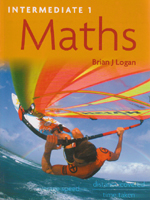Intermediate 1 Maths

By Brian J. Logan
October 2007
Distributed By Trans-Atlantic Publications, Inc.
Hodder Gibson
ISBN: 9780340939239345 Pages, Illustrated
\$45.00 Paper Original
Ages 15-17

Summary:

Intermediate 1 Maths offers an attractive and motivating textbook designed to enthuse students. Attractively illustrated with colour coded explanations and worked examples, the book brings Maths into real-life contexts with activities that make the subject relevant to Intermediate 1 students, whilst offering comprehensive coverage of the syllabus in a focused and concise manner.

There is an extensive range of relevant exam-style questions and assessment items to prepare for examination. A CD is included with the book, containing answers to all of the student exercises. The disc can be retained for use solely by the teacher, or released for student self-access according to preferred teaching and assessment strategies.

The book covers all the outcomes in the four units of the Intermediate 1 Mathematics course, i.e. units 1, 2, 3 and the Applications of Mathematics unit. Each chapter concentrates on the required learning outcomes with questions, exercises, and end of topic summaries. Questions are designed to help students prepare and practise for unit tests, and the final exam features prominently throughout the book, whilst students are directed to the relevant chapters of the course depending on which units they are studying.

Attention is given to both calculator and non-calculator examples in line with the two papers which make up the final exam, and each chapter contains student exercises (with answers supplied). A summary appears at the end of each chapter telling students what they should be able to do.

* A full colour, attractively designed textbook designed to motivate Intermediate 1 students
* A concise but comprehensive book for the fastest-growing syllabus area in Scottish education
* The latest in Hodder Gibson's immensely successful series of Intermediate 1 textbooks
* Includes a CD containing answers to all of the student exercises

UNIT 1
Chapter 1: Basic Calculations
Chapter 2: Basic Geometry
Chapter 3: Expressions and Formulae
Chapter 4: Everyday calculations: Earnings and Spending
End of Unit Tests

UNIT 2
Chapter 5: Integers
Chapter 6: Speed, Distance and Time
Chapter 7: The Theorem of Pythagoras
Chapter 8: Graphs, Charts and Tables
Chapter 9: Using Simple Statistics
End of Unit Tests

UNIT 3
Chapter 10: Simple Algebraic Operations
Chapter 11: Graphical Relationships
Chapter 12: Trigonometry in a Right-Angled Triangle
Chapter 13: Standard Form
End of Unit Tests

Applications of Mathematics Unit
Chapter 14: Social Arithmetic: Earnings and Borrowing
Chapter 15: Logic Diagrams
Chapter 16: Applications of Geometry
Chapter 17: Statistical Assignment
End of Unit Tests

PRACTICE EXAM PAPER(Papers 1 and 2)